## 10/28/2023

### 2 to the power of 0 to 1002 to the power of 0 to 29

The successive powers of two from 0 to 29 are presented in the table above. The table of powers of the number 2 begins with the exponent zero. Any number to the zero power is equal to one. Therefore, 2 to the power of 0 is equal to 1. Any number to the first power is equal to itself. Therefore, 2 to the power of 1 is equal to 2.

If this table is not enough for someone, then you can look at another one, where the powers of the number 2 are presented up to the 100th power.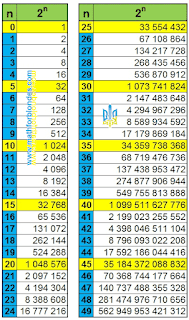2 to the power of 0 to 49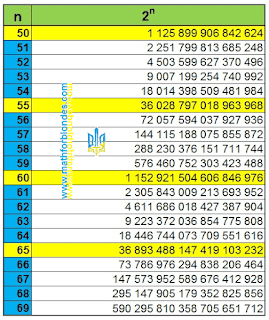2 to the power of 50 to 692 to the power of 70 to 892 to the power of 90 to 100

I hope programmers will like these tables of powers of the number 2 from 0 to 100. Mathematicians love to stick all sorts of nasty things anywhere. As a worthy student, I could not resist inserting 2 to the power “pi” and 2 to the power “e” into the table. Perhaps one of the child prodigies will find this useful. And now a little piece of theory.

Two to the second power means that the number two must be multiplied by itself. Therefore, 2 to the power of 2 or 2 squared equals four.

## 22 = 2 x 2 = 4

In general, the exponent shows how many identical numbers are multiplied together. So, two to the third power or 2 cubed means that three 2s are multiplied at the same time and this equals eight:

## 23 = 2 x 2 x 2 = 8

Two to the fourth power is equal to four twos multiplied:

## 24 = 2 x 2 x 2 x 2 = 16

This table of successive powers of the number 2 is very often used in programming, since it uses the binary number system.

## 10/18/2023

### Negative numbers: numbers game

You have all seen the game of chess. Adults go on stage, sit at a table opposite each other and start the game.

Let's organize a similar numbers game between two mathematicians. The rule is very simple - the mathematician who correctly depicts the number axis wins.

There is a piece of paper between the players. There is already ONE straight line drawn on the sheet. The players' task is to turn this line into a number axis. Players sit down at the table and start the game.

Players draw a zero, place an arrow in the direction of positive numbers, and choose the size of one unit. After this, positive numbers and negative numbers appear on the playing field. Game over.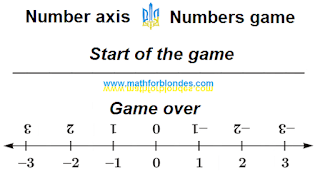Numbers axis. Numbers game.

The game ended in a draw. Both mathematicians drew the number axis correctly, as they were taught. Further, the winner can be determined by the number of scientific publications or through a boxing match.

What did we get? All numbers are both positive and negative at the same time. The sign of the number depends on which side we look at the number аxis.

The secret is that in nature numbers have no sign. The plus and minus signs are a reflection of our own opinion about a particular number. This opinion may be different for two different people.

## 10/17/2023

### Negative numbers: it's debtAuthor Nikolay Khyzhnjak

You can't take life from the dead.

In one popular science film, a mathematician gave an example about negative numbers. "You have minus one fish. You went fishing, you caught one fish and you have nothing left. Amazing result!".

The mathematical record of this story looks like this:

## -1 + 1 = 0

Now suppose I am a fisherman. This is how I see this story. Someone says that I have minus one fish. I don't have anything. I write zero.

## 0

I go fishing and I catch one fish. Now I have one fish. I add one.

## 0 + 1 = 1

After that, the one who claimed that I have minus one fish appears and takes my fish for himself.

## 0 + 1 - 1 = 0

Ups! Where are the negative numbers here? This is an ordinary racket.

Now let's look at what it means to borrow. I urgently need money, 1 dollar. What do I have now? Nothing, zero.

## 0

A good man (or an evil bank) gave me \$1. What do I have now? I have a real, positive \$1 that I can spend however I want. No, I won't waste it. I'll keep it as a museum piece.

## 0 + 1 = 1

After that I earned 1 dollar. How much money do I have?

## 1 + 1 = 2

I am an honest person and I pay my debts. How much money will I have left?

## 2 - 1 = 1

I borrowed money, I paid it back and I was left with \$1. Or I will still have what I bought. What was it? Were these negative numbers? No. It was a time machine that allows me to buy today what I can earn only tomorrow.

The main rule is this: it is impossible to borrow something that does not exist. Try borrowing a time travel machine, a perpetual motion machine, or a flying saucer. This is impossible to do because these things do not exist. And no matter how many minus signs you promise, you still won’t get these things. You can't take life from the dead.

Next, I suggest playing a numbers game.

### Table of primes

A prime number (or a prime) is a natural number greater than 1 that is not a product of two smaller natural numbers. Prime numbers have exactly two divisors: 1 and the number itself. Below is a table of prime numbers up to 20000.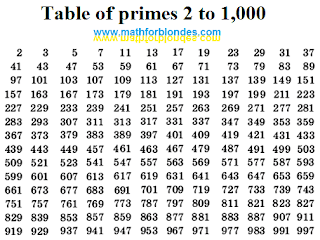Table of primes 2 to 1,000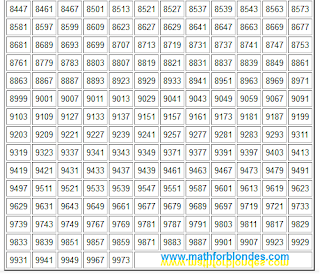Table of primes 1,000 to 10,000Table of primes 10,000 to 15,000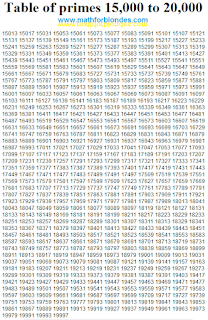Table of primes 15,000 to 20,000

Prime numbers are a favorite toy of mathematicians. As of January 2019, the largest prime number known to mathematicians had 24,862,048 decimal digits. This prime numbers game is so addicting that even I am addicted to this game. What can I say about this? Playing prime numbers is very time-consuming and tedious, but the results can be very interesting. I came up with my own rules of the game, which I will tell you about soon. I hope this will be of interest to mathematicians.

I needed prime numbers up to 20,000. Here you will find prime numbers up to 21,000,000.